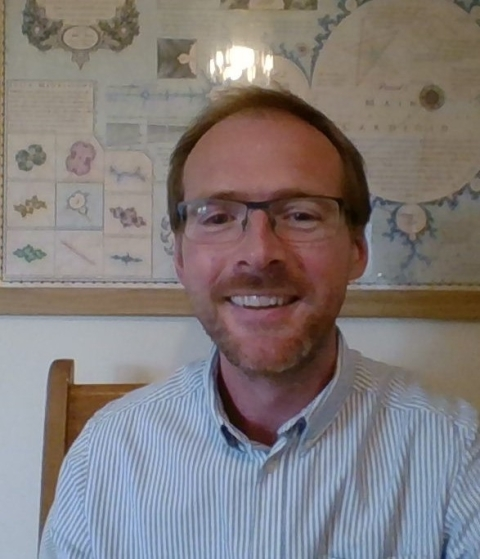PhD Supervisor

## Biography

I am currently Associate Head (Curriculum) in Mathematics in the School of Mathematics and Physics (formerly the Department of Mathematics) where I have worked since 2010. Previously, I was a lecturer in the Department of Mathematical Physics at the National University of Ireland, Galway; a Post-Doctoral Fellow at the University of Strathclyde, Glasgow; and a PhD student at Dublin City University, Ireland.

My PhD thesis title was “Stability of naked singularities in self-similar collapse” (Supervisor, Dr Brien Nolan, awarded 2006) and was in the area of General Relativity. At Strathclyde I worked with Professor McInnes on a project titled “Dynamics, stability and control of highly non-Keplerian orbits” which was in the area of Astrodynamics (specifically solar sails). Since then I have also become interested in questions of integrability of Hamiltonian systems, with particular emphasis on geodesic flow, and the envelopes of geodesics and lines in curved spaces (the so-called "conjugate locus").

## Research interests

The main topic of current research is the focusing of geodesics in surfaces and manifolds.

Geodesics are the straight lines of curves surfaces, a good example being the lines of longitude on a sphere. If we picture the lines of longitude emanating from the north pole of the sphere, they will come together at the south pole. However if we were to deform the sphere then the geodesics emanating from a point will not come together at another point, but will instead focus along a curve. This curve is called the conjugate locus but is also known as the caustic/envelope/focal set of geodesics. The study of the conjugate locus is complicated but rich, and uses notions from Differential Geometry, Singularity Theory, and Hamiltonian Dynamics.

Another topic of interest is the integrability of geodesic flows. The equations which define the geodesics in a surface or manifold have a Hamiltonian formulation, and a natural question is whether these equations can be “solved” in some sense (Liouville integrability). Recent work has shown that even seemingly simply surfaces have non-integrable geodesic equations which implies the long-time behaviour of these ‘straight line’ curves can be irregular and chaotic.

Finally a topic from Astrodynamics: the circular restricted 3-body problem admits a number of equilibrium points and emanating from these are families of periodic orbits. An interesting extension is to consider a solar sail in the Earth-Sun system (for example ) and ask what are the periodic orbits available to it, what are their stability and connectedness properties, and how do they differ from the classical case. Methods used involve continuation of periodic orbits and techniques from Bifurcation Theory.